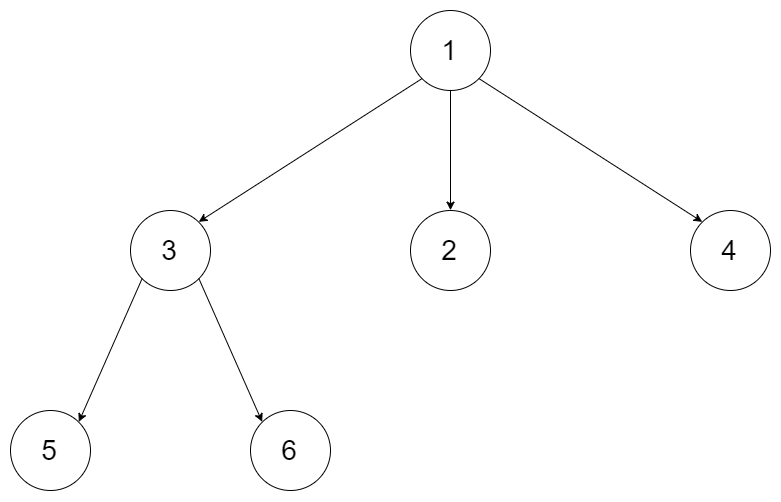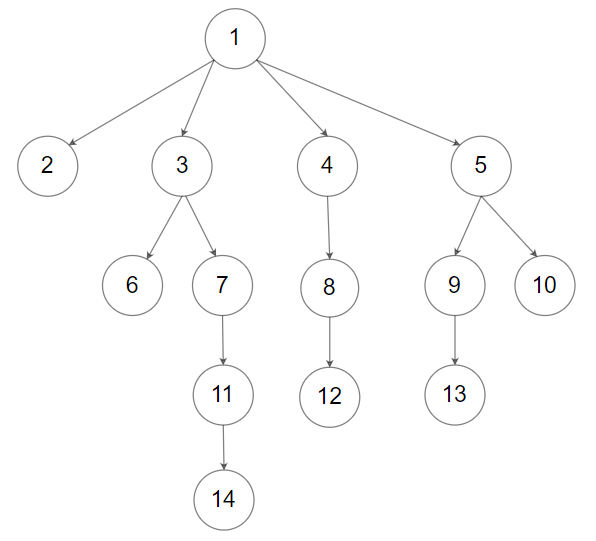# 559. Maximum Depth of N-ary Tree

Maximum Depth of N-ary Tree - LeetCode Given a n-ary tree, find its maximum depth. The maximum depth is the number of nodes along the longest path from the root node down to the farthest leaf node. Nary-Tree input serialization is represented in their level order traversal, each group of children is separated by the null value (See examples).

Example 1:Input: root = [1,null,3,2,4,null,5,6] Output: 3 Example 2:Input: root = [1,null,2,3,4,5,null,null,6,7,null,8,null,9,10,null,null,11,null,12,null,13,null,null,14] Output: 5

Constraints:

The total number of nodes is in the range [0, 104]. The depth of the n-ary tree is less than or equal to 1000.

• code
``````class Solution {
public int maxDepth(Node root) {
if (root == null) return 0;
int depth = 0;
for (Node node: root.children){
depth = Math.max(depth, maxDepth(node));
}
return depth + 1;
}
}
``````
• code
``````class Solution:
def maxDepth(self, root: 'Node') -> int:
if not root: return 0
depth = 0
q = deque([root])
while q:
depth += 1
for i in range(len(q)):
cur = q.popleft()
if cur:
for child in cur.children:
q.append(child)
return depth
``````